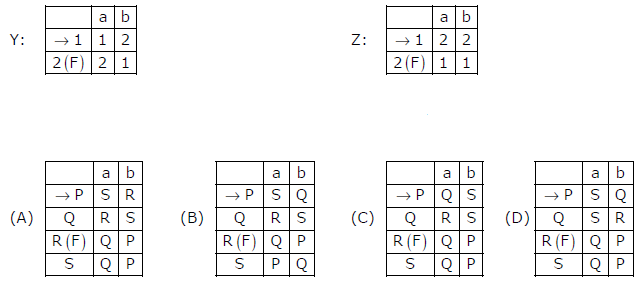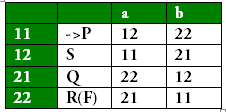# GATE | GATE CS 2008 | Question 49

Given below are two finite state automata (→ indicates the start state and F indicates a final state)Which of the following represents the product automaton Z×Y?(A) A
(B) B
(C) C
(D) D

Explanation: All four states 11, 21, 22 , 12 are interpreted as P, Q, R and S. By looking at options we can easily find out that →11 is P and 22(F) is R. Now say 12 is S and 21 is Q.
Let’s construct the transition table for ZxY.So, the answer should be (A) but in the row for S, it should be P and Q and not Q and P.

Please comment below if you find anything wrong in the above post.

Quiz of this Question